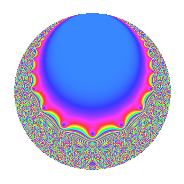# Properties

 Label 5.10.bLevel 5 Weight 10 Character orbit b Rep. character $$\chi_{5}(4,\cdot)$$ Character field $$\Q$$ Dimension 4 Newform subspaces 1 Sturm bound 5 Trace bound 0

# Related objects

## Defining parameters

 Level: $$N$$ = $$5$$ Weight: $$k$$ = $$10$$ Character orbit: $$[\chi]$$ = 5.b (of order $$2$$ and degree $$1$$) Character conductor: $$\operatorname{cond}(\chi)$$ = $$5$$ Character field: $$\Q$$ Newform subspaces: $$1$$ Sturm bound: $$5$$ Trace bound: $$0$$

## Dimensions

The following table gives the dimensions of various subspaces of $$M_{10}(5, [\chi])$$.

Total New Old
Modular forms 6 6 0
Cusp forms 4 4 0
Eisenstein series 2 2 0

## Trace form

 $$4q - 1368q^{4} + 1140q^{5} + 2808q^{6} + 11628q^{9} + O(q^{10})$$ $$4q - 1368q^{4} + 1140q^{5} + 2808q^{6} + 11628q^{9} - 69160q^{10} + 109968q^{11} - 424536q^{14} - 396720q^{15} + 1631264q^{16} - 636880q^{19} - 3302280q^{20} + 3523968q^{21} - 2435040q^{24} - 1337900q^{25} + 6618768q^{26} - 3531720q^{29} + 3712680q^{30} - 10587712q^{31} + 26434624q^{34} + 13629840q^{35} - 56399976q^{36} + 1686816q^{39} + 43578400q^{40} - 16788552q^{41} + 20638944q^{44} + 55737180q^{45} - 61250072q^{46} - 46921028q^{49} - 150092400q^{50} + 84017088q^{51} + 115855920q^{54} - 26907120q^{55} + 315178080q^{56} - 460829040q^{59} - 307006560q^{60} + 360490568q^{61} + 134995072q^{64} + 183895680q^{65} + 18949536q^{66} - 286524864q^{69} - 508341960q^{70} - 47611872q^{71} + 1176861744q^{74} + 659239200q^{75} - 1168489440q^{76} - 728043520q^{79} + 965843040q^{80} - 343387836q^{81} + 1118898144q^{84} + 1275419840q^{85} - 2375904552q^{86} - 1582700760q^{89} - 1197088920q^{90} + 473322528q^{91} + 3327101704q^{94} + 1204791600q^{95} + 399339648q^{96} - 728787024q^{99} + O(q^{100})$$

## Decomposition of $$S_{10}^{\mathrm{new}}(5, [\chi])$$ into newform subspaces

Label Dim. $$A$$ Field CM Traces $q$-expansion
$$a_2$$ $$a_3$$ $$a_5$$ $$a_7$$
5.10.b.a $$4$$ $$2.575$$ 4.0.49740556.1 None $$0$$ $$0$$ $$1140$$ $$0$$ $$q+\beta _{1}q^{2}+(-\beta _{1}+\beta _{2})q^{3}+(-342+\cdots)q^{4}+\cdots$$